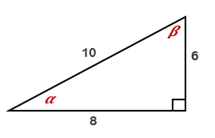Chapter 11.CT, Problem 2CT### Elementary Geometry for College St...

6th Edition
Daniel C. Alexander + 1 other
ISBN: 9781285195698

#### Solutions

Chapter
Section### Elementary Geometry for College St...

6th Edition
Daniel C. Alexander + 1 other
ISBN: 9781285195698
Textbook Problem
1 views

# For the right triangle shown, express each ratio as a fraction in lowest terms:a) cos Β______ b) sin α______To determine

a

To express:

The ratio cos β in the lowest term.

Explanation

Definition:

In a right triangle, the cosine ratio for an acute angle is the ratio adjacent sidehypotenuse.

Given:

The right triangle shown below

Calculation:

From the figure given,

For the angle β, adjacent side = 6 and hypotenuse = 10

To determine

b

To express:

The given sin α in the lowest term.

### Still sussing out bartleby?

Check out a sample textbook solution.

See a sample solution

#### The Solution to Your Study Problems

Bartleby provides explanations to thousands of textbook problems written by our experts, many with advanced degrees!

Get Started